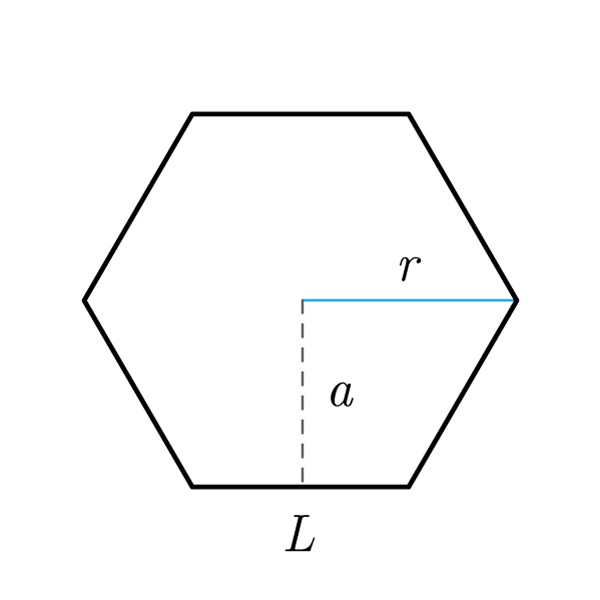# Hexagon

 Side $$S$$ Circumcircle's Radius $$R$$ Apothem $$a$$
$$2p = S \times 6$$
Perimeter
$$S = \frac{2p}{6}$$
$$A = \frac{2p \times a}{2}$$
Area
$$a = \frac{2A}{2p}$$
Apothem
$$2p = \frac{2A}{a}$$
Perimeter
Fixed number
$$f = 0.866 = \frac{a}{S}$$
Fixed number
$$a = S \times f$$
Apothem
$$S = \frac{a}{f}$$
Side
Area's costant
$$\varphi = 2.598 = \frac{A}{{S}^2}$$
Area's costant
$$A = {S}^2 \times \varphi$$
Area
$$S = \sqrt{\frac{A}{\varphi}}$$
Side
Inscribed Hexagon
$$S = R$$
Side
$$a = \frac{R \times \sqrt{3}}{2}$$
Apothem
$$A = \frac{ 3 \sqrt{3} \times {R}^2}{2}$$
Area
$$A = {R}^2 \times \varphi$$
Area
$$2p = 6 R$$
Perimeter

## Definition

A hexagon is a polygon with six sides. A regular hexagon is a regular polygon with six sides and six angles congruent.

## Properties

1. Polygon with six sides
2. The regular hexagon has six sides and six angles congruent, with a measure of 120°
3. A regular hexagon can be inscribed into a circle or circumscribed by a circle## Hexagon Formulas

Data Formula
Perimeter 2p = S × 6
Area A = (2p × a) / 2
Side S = 2p / 6
Fixed number f = 0.866 = a / S
Area's costant φ = 2.598 = A / (S2)
Apothem a = (2A) / (2p)
Perimeter 2p = (2A) / (a)
Apothem a = S × f
Side S = a / f
Area A = S2 × φ
Side S = √(A / φ)